## 利用简单的线性代数实现精确可控的窄带调色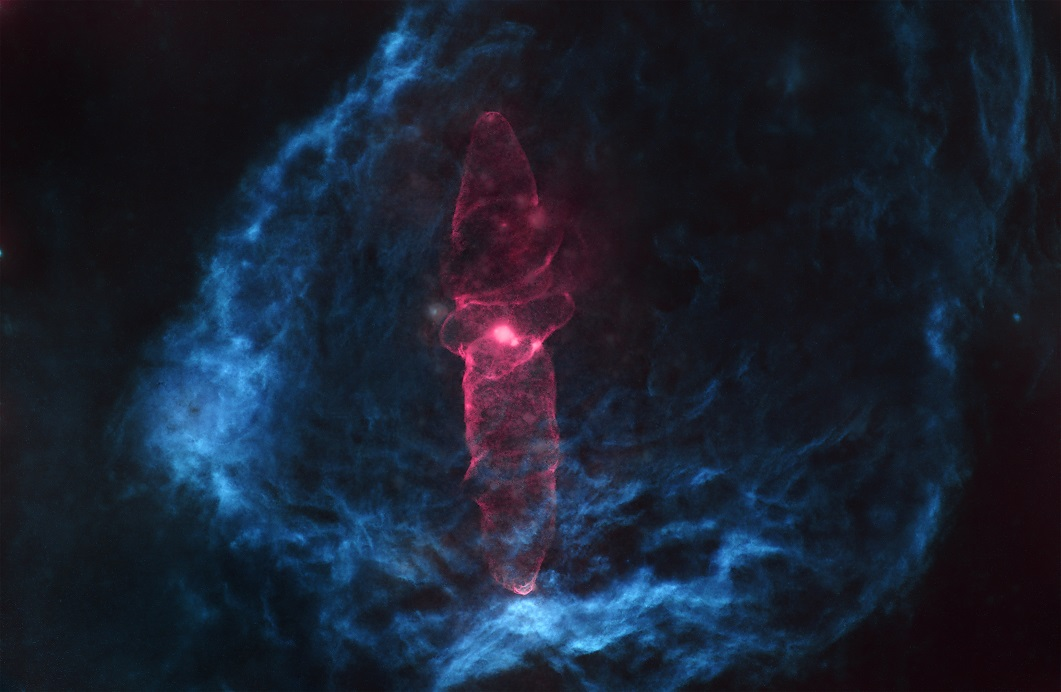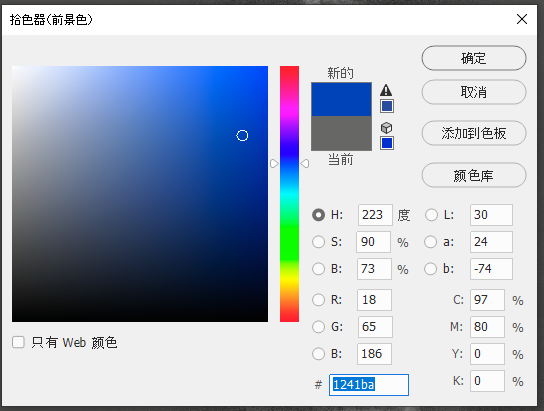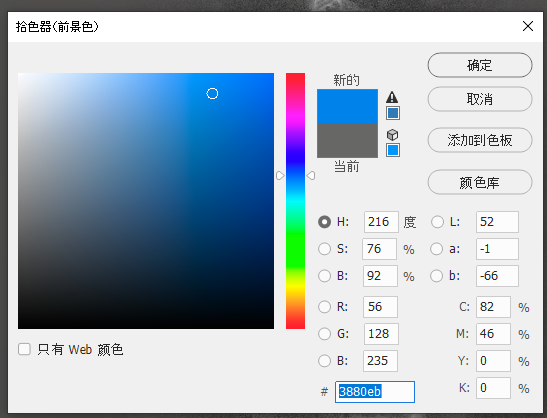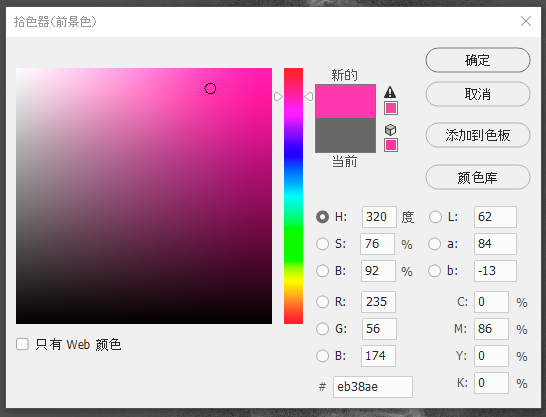R   G   B
H   18  65  186
S   56  128 235
O   235 56  174


• R: (18*H+56*S+235*O)/(18+56+235)
• G: (65*H+128*S+56*O)/(65+128+56)
• B: (186*H+235*S+174*O)/(186+235+174)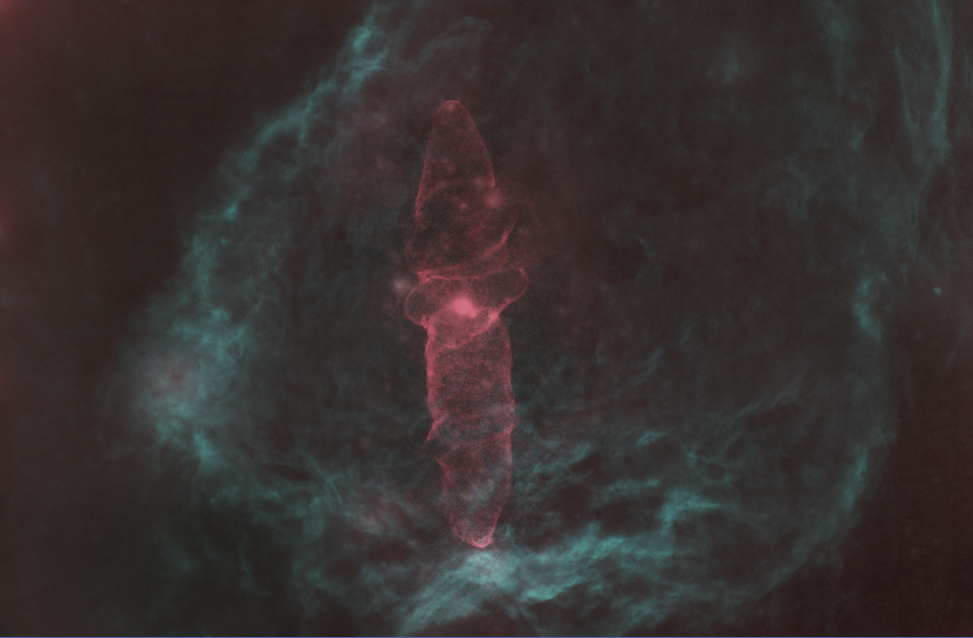$$\text{RGB}^T = R \cdot \text{HSO}^T$$

R是变换矩阵。或者如果我们把这个矩阵展开，就是：

$$\begin{bmatrix} R \ G \ B \end{bmatrix} = \begin{bmatrix} H_R & S_R & O_R \ H_G & S_G & O_G \ H_B & S_B & O_B \end{bmatrix} \cdot \begin{bmatrix}H \ S \ O\end{bmatrix}$$